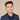# How To Filter An Array In Swift

Filtering an array is a common problem Swift developers face.

## How To Filter An Array In Swift With Code Examples

Let’s say you want to filter an array and only keep elements that are prefixed with “test”.

Here’s our string array example:

``let strArrayExample = ["test-1", "hello", "test-2", "blah blah", "test-3"]``

To get an array with only elements that are prefixed with “test”, we write the following code:

``let prefixedWithTest = strArrayExample.filter { \$0.hasPrefix("test") }``

Printing out `prefixedWithTest` will have the following output:

``["test-1", "test-2", "test-3"]``

You can also do the same with numbers. Here’s an example:

``let numbersArray = [-5, -2, -3, 1, 5, 100]``

Say we want to filter for only negative numbers:

``````let negative = numbersArray.filter { \$0 < 0 }
print(negative)``````

This will result in the following printed:

``[-5, -2, -3]``

You can make the condition to filter on anything. Here’s an example where we want only even numbers:

``````let even = numbersArray.filter { \$0 % 2 == 0 }
print(even)``````

Results in:

``[-2, 100]``

That’s how you filter arrays.

If you liked this post and want to learn more, check out The Complete iOS Developer Bootcamp. Speed up your learning curve - hundreds of students have already joined. Thanks for reading!## Eddy Chung

I teach iOS development on ZeroToAppStore.com.